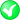#Boost C++ Libraries

...one of the most highly regarded and expertly designed C++ library projects in the world.

##### area

Calculates the area of a geometry.

###### Description

The free function area calculates the area of a geometry. It uses the default strategy, based on the coordinate system of the geometry.

The area algorithm calculates the surface area of all geometries having a surface, namely box, polygon, ring, multipolygon. The units are the square of the units used for the points defining the surface. If subject geometry is defined in meters, then area is calculated in square meters.

The area calculation can be done in all three common coordinate systems, Cartesian, Spherical and Geographic as well.

###### Synopsis

```template<typename Geometry>
area_result<Geometry>::type area(Geometry const & geometry)```

###### Parameters

Type

Concept

Name

Description

Geometry const &

Any type fulfilling a Geometry Concept

geometry

A model of the specified concept

###### Returns

The calculated area

Either

`#include <boost/geometry.hpp>`

Or

`#include <boost/geometry/algorithms/area.hpp>`

###### Conformance

The function area implements function Area from the OGC Simple Feature Specification.

###### Behavior

Case

Behavior

pointlike (e.g. point)

Returns 0

linear (e.g. linestring)

Returns 0

areal (e.g. polygon)

Returns the area

Cartesian

Returns the area in the same units as the input coordinates

Spherical

Returns the area on a unit sphere (or another sphere, if specified as such in the constructor of the strategy)

Reversed polygon (coordinates not according their orientiation)

Returns the negative area

###### Supported geometries

Geometry

Status

PointSegmentBoxLinestringRingPolygonMultiPointMultiLinestringMultiPolygonVariantLinear

###### Examples

Calculate the area of a polygon

```#include <iostream>

#include <boost/geometry.hpp>
#include <boost/geometry/geometries/point_xy.hpp>
#include <boost/geometry/geometries/polygon.hpp>

namespace bg = boost::geometry;int main()
{
// Calculate the area of a cartesian polygon
bg::model::polygon<bg::model::d2::point_xy<double> > poly;
bg::read_wkt("POLYGON((0 0,0 7,4 2,2 0,0 0))", poly);
double area = bg::area(poly);
std::cout << "Area: " << area << std::endl;

// Calculate the area of a spherical equatorial polygon
bg::model::polygon<bg::model::point<float, 2, bg::cs::spherical_equatorial<bg::degree> > > sph_poly;
bg::read_wkt("POLYGON((0 0,0 45,45 0,0 0))", sph_poly);
area = bg::area(sph_poly);
std::cout << "Area: " << area << std::endl;

return 0;
}
```

 Convenient namespace alias

Output:

```Area: 16
Area: 0.339837
```